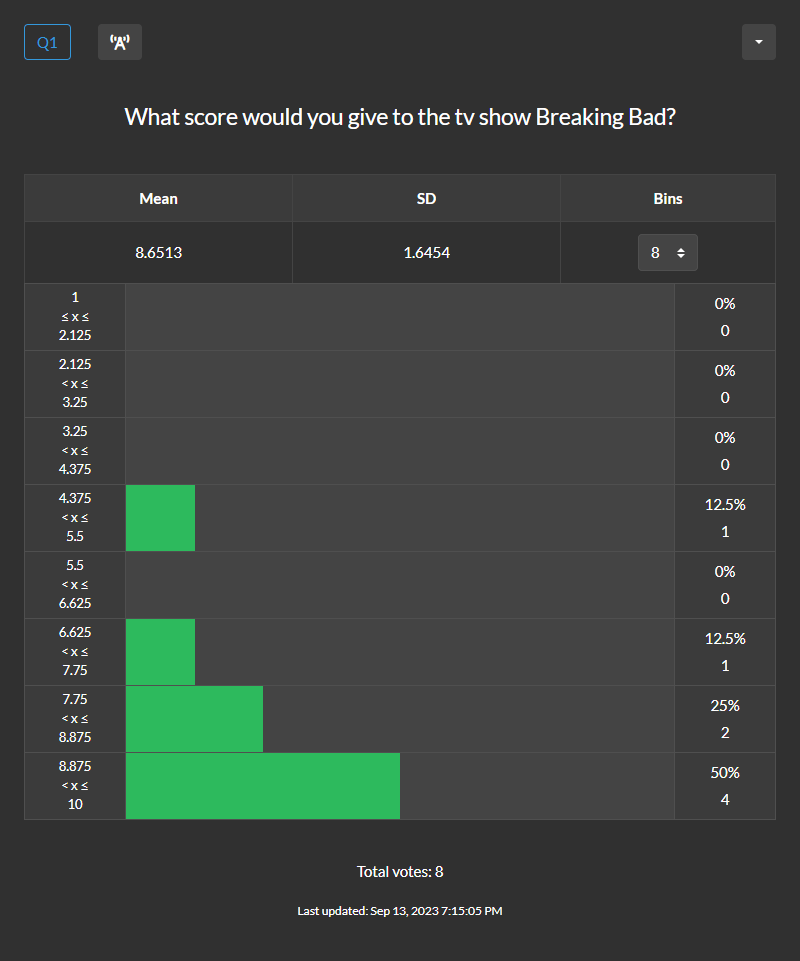# Types of Questions

Each poll you create must have at least 1 question. Each question can be text or number based. For text questions each option is a text value. We have 5 types of text questions. This includes single choice, multiple choice, range, ranked and probability. For number questions each option is a numeric value. We have 2 types of number questions. This includes integer and rational.

##### Single choice

This is also known as plurality voting, first past the post and relative majority. This is a type of text question. Each option is a text value. Voters select 1 of the options. You can also give voters the ability to write in their own option.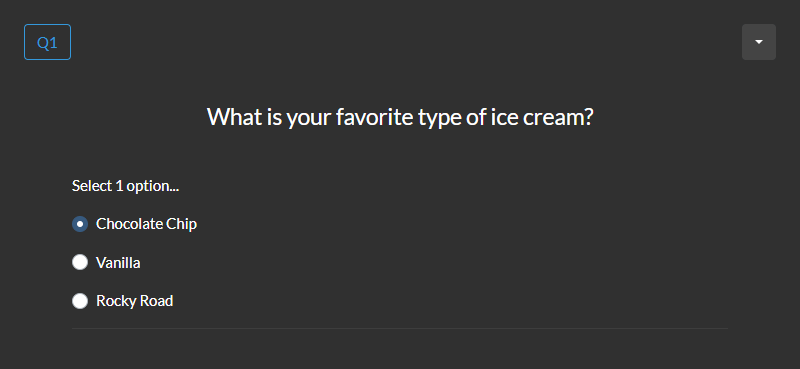The results show the score for each option. Percent stats are also calculated. Score is the number of times an option was selected. Percent is score divided by total votes.##### Multiple choice

This is also known as approval voting. This is a type of text question. Each option is a text value. Voters can select multiple options based on constraints defined by the poll creator.The results show the score for each option. Support and percent stats are also calculated. Score is the number of times an option was selected. Support is score divided by total votes. For example, a question has A and B as options. A is selected in the first vote. A and B are selected in the second vote. A is selected in 100% of the votes. B is selected in 50% of the votes. Percent is score divided by total points. For example, a question has A and B as options. A is selected in the first vote. A and B are selected in the second vote. A has 2 points. B has 1 point. There are 3 total points. A has 67% of the points. B has 33% of the points.##### Range

This is also known as score voting, utility voting and interval measure voting. This is a type of text question. Each option is a text value. Voters give each option a score between a min and max defined by the poll creator.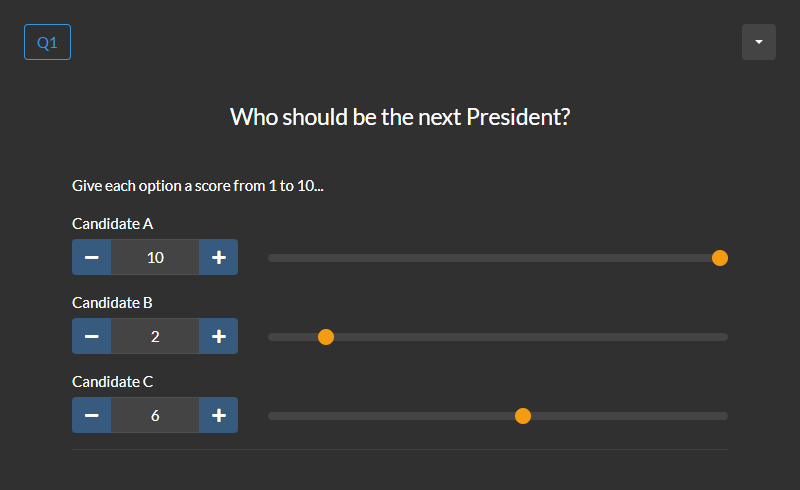The results show the score for each option. Average score, support and percent stats are also calculated. Score is the number of points an option has received. Average score is score divided by total votes. This is the average number of points an option gets per vote. Support is score divided by max points possible. For example, a question has A and B as options. Each option can get up to 10 points per vote. A gets 8 points and B gets 4 points in the first vote. A has 80% of the max points possible. B has 40% of the max points possible. Percent is score divided by total points. For example, a question has A and B as options. A gets 2 points and B gets 1 point in the first vote. There are 3 total points. A has 67% of the points. B has 33% of the points.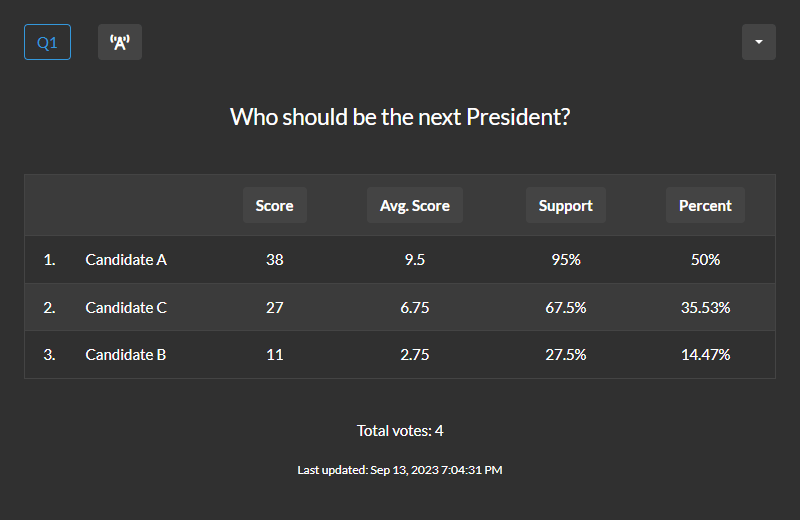##### Ranked

This is also known as the Borda count. This is a type of text question. Each option is a text value. Voters rank the options from first to last. For each vote, an option receives points based on its position and the total number of options (n). The option in the first position gets n points. The option in the second position gets n - 1 points. This pattern continues for each position. The option in the last position gets 1 point.The results show the score for each option. Average rank and percent stats are also calculated. Score is the number of points an option has received. For example, a question has A, B and C as options. The ranking is C, A, B in the first vote. A has 2 points. B has 1 point. C has 3 points. Average rank is the average position of an option per vote. A lower number means a higher rank. Percent is score divided by total points. For example, a question has A, B and C as options. The ranking is B, A, C in the first vote. A has 2 points. B has 3 points. C has 1 point. There are 6 total points. A has 33% of the points. B has 50% of the points. C has 17% of the points.##### Probability

This is also known as odds voting. This is a type of text question. Each option is a text value. Voters distribute 100 points based on the probability of each option. Options should be mutually exclusive. This means only 1 of the options will occur.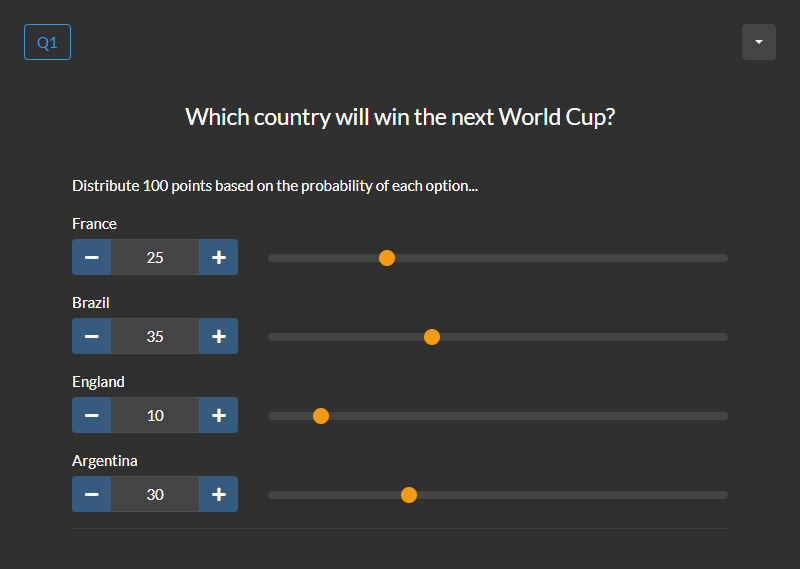The results show the score for each option. The odds and percent stats are also calculated. Score is the number of points an option has received. Odds is a positive or negative number at least 3 digits in length. A negative value means a probability greater than 50%. A positive value means a probability less than or equal to 50%. Percent is score divided by total points. This is the average probability an option gets per vote.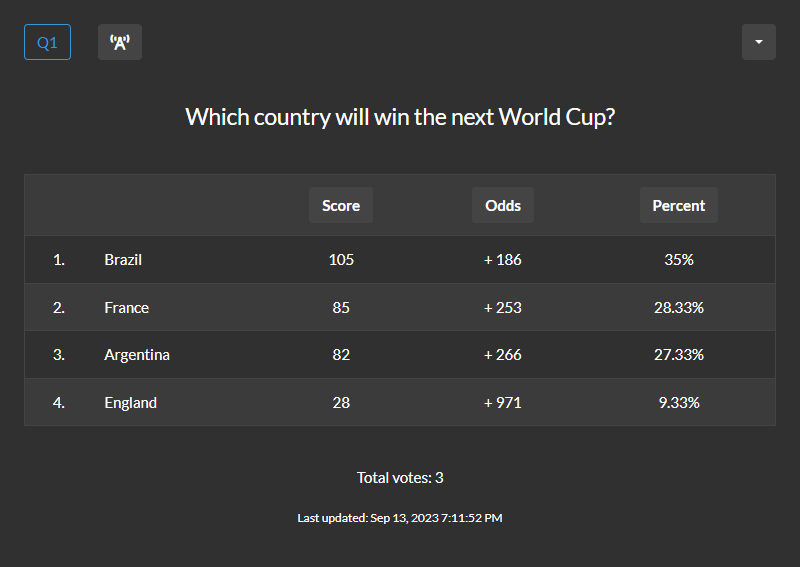##### Integer

This is a type of number question. Each option is a numeric value. Voters select an integer between a min and max defined by the poll creator. Integer questions allow for a smaller range of numbers than rational questions. If you need a larger range of numbers try a rational question.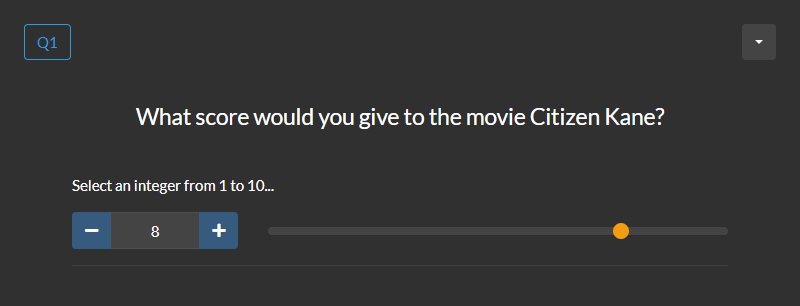The results show the score for each number. Mean, median, mode, standard deviation and percent stats are also calculated. Integer questions can be added to a list. Read the how to create a list guide to learn more.##### Rational

This is a type of number question. Each option is a numeric value. Voters select a rational number between a min and max defined by the poll creator.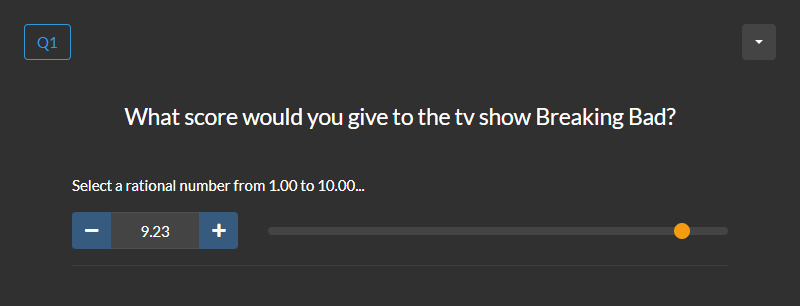The results show the score for each bin of numbers. Mean, standard deviation and percent stats are also calculated. Rational questions can be added to a list. Read the how to create a list guide to learn more. Rational questions have less descriptive results than integer questions. If you need more descriptive results try an integer question.